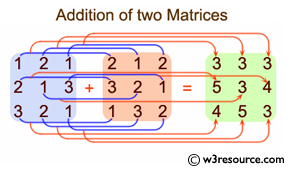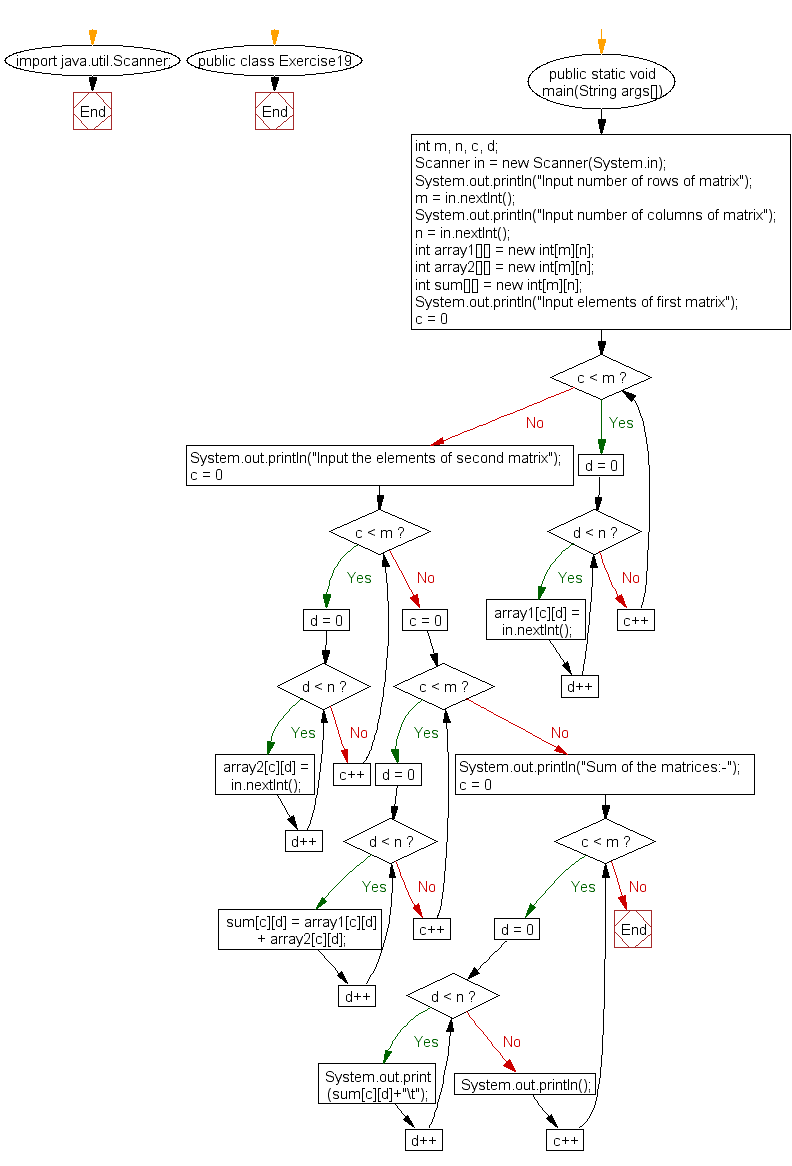﻿ Java exercises: Add two matrices of the same size - w3resource# Java Array Exercises: Add two matrices of the same size

## Java Array: Exercise-19 with Solution

Write a Java program to add two matrices of the same size.

Pictorial Presentation:Sample Solution:

Java Code :

``````import java.util.Scanner;
public class Exercise19 {
public static void main(String args[])
{
int m, n, c, d;
Scanner in = new Scanner(System.in);

System.out.println("Input number of rows of matrix");
m = in.nextInt();
System.out.println("Input number of columns of matrix");
n  = in.nextInt();

int array1[][] = new int[m][n];
int array2[][] = new int[m][n];
int sum[][] = new int[m][n];

System.out.println("Input elements of first matrix");

for (  c = 0 ; c < m ; c++ )
for ( d = 0 ; d < n ; d++ )
array1[c][d] = in.nextInt();

System.out.println("Input the elements of second matrix");

for ( c = 0 ; c < m ; c++ )
for ( d = 0 ; d < n ; d++ )
array2[c][d] = in.nextInt();

for ( c = 0 ; c < m ; c++ )
for ( d = 0 ; d < n ; d++ )
sum[c][d] = array1[c][d] + array2[c][d];

System.out.println("Sum of the matrices:-");

for ( c = 0 ; c < m ; c++ )
{
for ( d = 0 ; d < n ; d++ )
System.out.print(sum[c][d]+"\t");

System.out.println();
}
}
}
```
```

Sample Output:

```Input number of rows of matrix
2
Input number of columns of matrix
2
Input elements of first matrix
1
2
3
4
Input the elements of second matrix
5
6
7
8
Sum of the matrices:-
6       8
10      12
```

Flowchart:Java Code Editor:

Improve this sample solution and post your code through Disqus

What is the difficulty level of this exercise?

Test your Programming skills with w3resource's quiz.

﻿

## Java: Tips of the Day

countOccurrences

Counts the occurrences of a value in an array.

Use Arrays.stream().filter().count() to count total number of values that equals the specified value.

```public static long countOccurrences(int[] numbers, int value) {
return Arrays.stream(numbers)
.filter(number -> number == value)
.count();
}
```

Ref: https://bit.ly/3kCAgLb